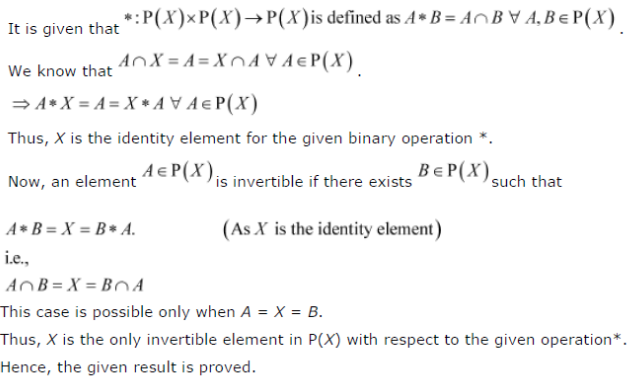# Given a non-empty set X, consider the binary operation * : P (X) x P (X) ∀ P (X)

Given a non-empty set X, consider the binary operation * : P (X) x P (X) ∀ P (X) given by A * B = A ∀ B ∀ A, B in P (X), where P (X) is the power set of X. Show that X is the identity element for this operation and X is the only invertible element in P (X) with respect to the operation *.